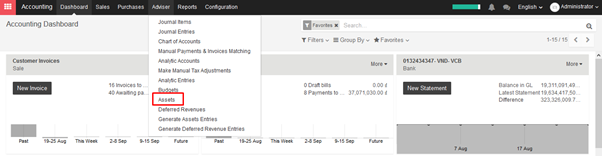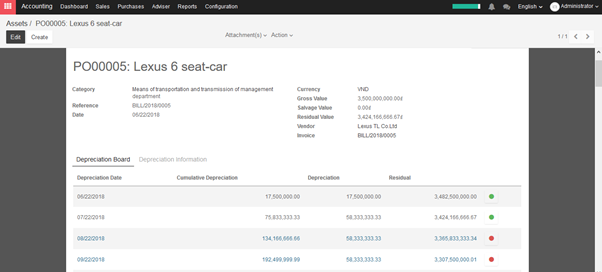# 7.8.4. Depreciate an Asset

→ Assets depreciation generate automatically from Vendor bills

To depreciate an asset, open Accounting module ‣ Adviser ‣ AssetsKIU will create depreciation journal entries automatically at the right date for every confirmed asset. (not the draft ones). You can control in the depreciation board: a green bullet point means that the journal entry has been created for this line.

But you can also post journal entries before the expected date by clicking on the green bullet and forcing the creation of related depreciation entry.In the Depreciation board, click on the red bullet to post the journal entry. Click on the Items button on the top to see the journal entries which are already posted.

→ Create assets depreciation manually

To register an asset manually, go to the menu Adviser ‣ Assets.

Once your asset is created, don’t forget to Confirm it. You can also click on the Compute Depreciation button to check the depreciation board before confirming the asset.

Note:

If you create asset manually, you still need to create the supplier bill for this asset. The asset document will only produce the depreciation journal entries, not those related to the supplier bill.

Explanation of the fields:

Status

When an asset is created, the status is ‘Draft’.

If the asset is confirmed, the status goes in ‘Running’ and the depreciation lines can be posted in the accounting.

You can manually close an asset when the depreciation is over. If the last line of depreciation is posted, the asset automatically goes in that status.

Category

Category of asset

Date

Date of asset

Gross Value

Gross value of asset

Salvage Value

It is the amount you plan to have that you cannot depreciate.

Computation Method

Choose the method to use to compute the amount of depreciation lines.

* Linear: Calculated on basis of: Gross Value / Number of Depreciations

* Degressive: Calculated on basis of: Residual Value * Degressive Factor

Time Method

Choose the method to use to compute the dates and number of depreciation lines.

* Number of Depreciations: Fix the number of depreciation lines and the time between 2 depreciations.

* Ending Date: Choose the time between 2 depreciations and the date the depreciations won’t go beyond.

Prorata Temporis

Indicates that the first depreciation entry for this asset have to be done from the purchase date instead of the first January / Start date of fiscal year

Number of Depreciations

The number of depreciations needed to depreciate your asset

Number of Months in a Period

The amount of time between two depreciations, in months